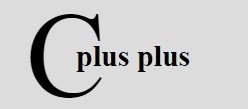# C++ Basic Syntax

« Previous Tutorial Next Tutorial »

To understand basic syntax of any C++ program. Let's look at this basic and general form of a simple C++ program.

```/* Multi-line comments */

return_type main()
{
clrscr();
program_code_statements;
getch();
}```

This is the basic syntax of a simple C++ program. In the above code fragment, the function, clrscr() is used to clear the output screen. Whereas the function getch() holds the output screen until user press a key.

## Semicolons in C++

Semicolons in C++ are used to terminate statements. Here is an example of semicolons in C++:

```int num;
int sum = 0;
fun(void);```

## Block in C++

A block in C++, basically is a set of logically connected statements inside opening and closing braces. Here is an example:

```{
cout << "Hello C++, I am Block";
return 0;
}```

## Whitespace in C++

Whitespaces may be blanks, newlines, tabs, etc. Here is an example. Here there is a whitespace between the type int and variable age:

`int age;`

Now let's take some example programs from simple to function program to understand the basic syntax of a simple C++ program. Here we have provided 7 type of C++ programs for understanding the basic syntax of these programs. Let's start with simplest C++ program, which will print "Hello, World" on the output screen.

```/* C++ Basic Syntax */

#include<iostream.h>
#include<conio.h>
void main()
{
clrscr();
cout<<"Hello, World";
getch();
}```

Here is the sample output of this C++ program:

Here is another type of C++ program, uses if statement:

```/* C++ Basic Syntax */

#include<iostream.h>
#include<conio.h>
void main()
{
clrscr();
char ch;
cout<<"Press y to see greeting: ";
cin>>ch;
if(ch=='y' || ch=='Y')
{
cout<<"\nWelcome to codescracker.com";
}
getch();
}```

Here is the sample run of the above C++ program:

Here is one more type of C++ program, uses if-else statement:

```/* C++ Basic Syntax */

#include<iostream.h>
#include<conio.h>
void main()
{
clrscr();
char ch;
cout<<"Press y to see greeting: ";
cin>>ch;
if(ch=='y' || ch=='Y')
{
cout<<"\nWelcome to codescracker.com";
}
else
{
cout<<"\nPress any key to exit..\n";
}
getch();
}```

Below are the two sample run of the above C++ program:

Let's take another type of C++ program, uses for loop:

```/* C++ Basic Syntax */

#include<iostream.h>
#include<conio.h>
void main()
{
clrscr();
int i;
for(i=0; i<10; i++)
{
cout<<"*  ";
}
getch();
}```

Below is the sample output of this C++ program:

Here is another type of C++ program, uses while loop

```/* C++ Basic Syntax */

#include<iostream.h>
#include<conio.h>
void main()
{
clrscr();
int i=0;
while(i<10)
{
cout<<"*  ";
i++;
}
getch();
}```

Below is the sample output of the above C++ program. This output is same as the above one.

Below is another type of C++ program, uses do-while loop:

```/* C++ Basic Syntax */

#include<iostream.h>
#include<conio.h>
void main()
{
clrscr();
int i=0;
do
{
cout<<"*  ";
i++;
}while(i<10);
getch();
}```

Here is the sample output of the above C++ program. This C++ program also produces the same output as the above two C++ program produced.

Let's take one more type of C++ program, uses function.

```/* C++ Basic Syntax */

#include<iostream.h>
#include<conio.h>
void greet(void);
void main()
{
clrscr();
cout<<"Press any key for greeting..";
getch();
greet();
getch();
}
void greet()
{
cout<<"\n\nWelcome to codescracker.com";
}```

Below is the sample run of the above C++ program:

### More Examples

Here are some more C++ programs listed, that you can go for:

C++ Online Test

« Previous Tutorial Next Tutorial »